Fourth-order Runge-Kutta Method using Mathematica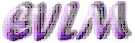Аnotation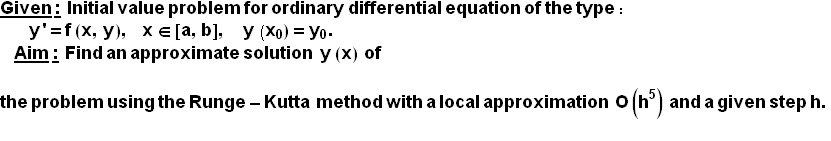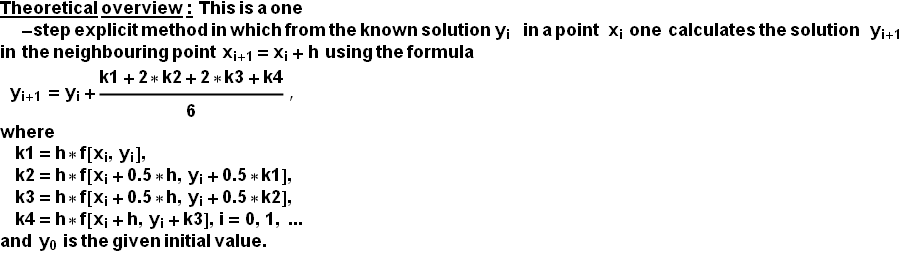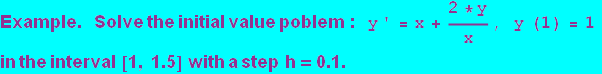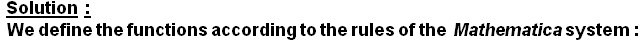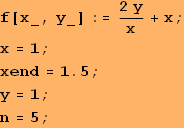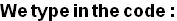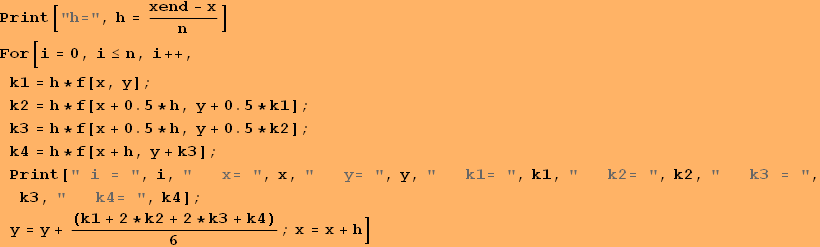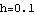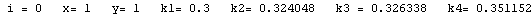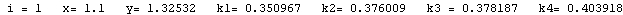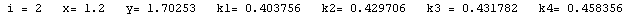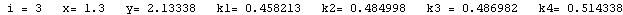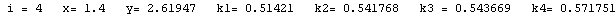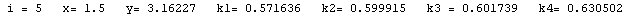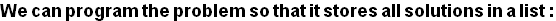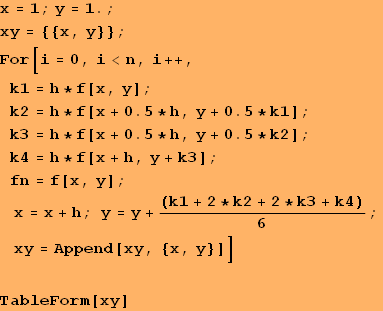1 1 1.1 1.32532 1.2 1.70253 1.3 2.13338 1.4 2.61947 1.5 3.16227

Conclusion:   The solution derived using the Runge-Kutta method O(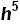) is in the form of a table of the variable function y(x), shown in the second column of the table above.  Since the  local apriori error of the method is O() (or global error is  O(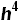) ) and here h=0.1, then these solutions must be rounded to five symbols after the decimal point.

Graphic of the solution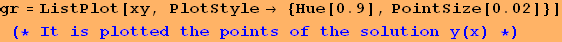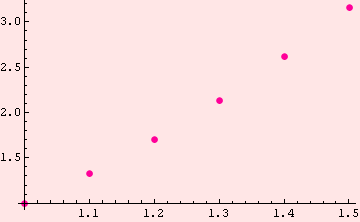Created by Wolfram Mathematica 6.0  (22 September 2008)